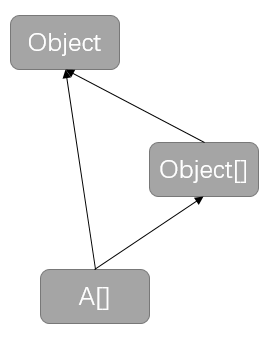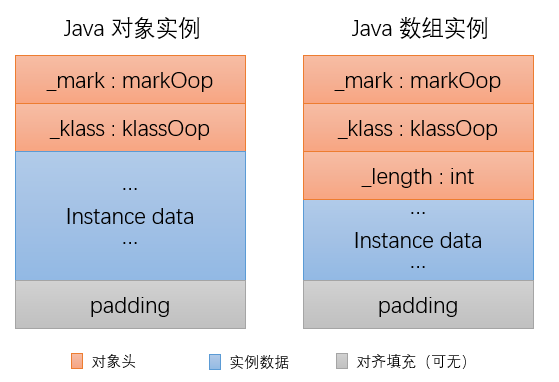JDK源码分析（2）之 Array 相关

一、Array 是一个是对象吗？

• 数组是协变的，所以Object[]A[]的父类，即Object[] o = A[];
• 数组是一个对象，所以数组的父类是Object，即Object oo = o;
• 那么A[]Object[]Object是什么关系呢？private static void test05() {
Object[] o = new String;
System.out.println(o.getClass().getName());
System.out.println(o.getClass().getSuperclass().getName());
String[] s = (String[]) o;
System.out.println(s.getClass().getSuperclass().getName());
Object oo = s;
}

[Ljava.lang.String;
java.lang.Object
java.lang.Object

extends关键字产生的继承关系是怎么定义呢？

// ClassFile 结构
ClassFile {
u4                magic;
u2                minor_version；
u2                major_version;
u2                constant_pool_count；
cp_info           constant_pool[constant_pool_count-1];
u2                access_flags;
u2                this_class;
u2                super_class;
u2                interfaces_count;
u2                interfaces[interfaces_count];
u2                fields_count;
field_info        fields[fields_count]；
u2                methods_count;
method_info       methods[methods_count];
u2                attribute_count;
attributes_info;  attributes[attributes_count]；
}

二、Array 的 length 域相关

• An array's length is not part of its type.
private static void test06() {
String[] s = new String;
System.out.println(s.length);
System.out.println(s.getClass().getDeclaredFields().length);
try {
System.out.println(s.getClass().getDeclaredField("length"));
} catch (NoSuchFieldException e) {
System.out.println(e.toString());
}
}

2
0
java.lang.NoSuchFieldException: length三、Array 的创建流程

// 数组创建的几种形式
String[] s = {"a", "b", "c"};  // 初始化器
String[] s1 = new String;   // 有维度表达式
String[] s2 = (String[]) Array.newInstance(String.class, 3); // 有维度表达式

无：
创建的时候每个元素递归深入初始化，失败则退出
变量类型检查 -> 与数组类型不兼容 -> 编译错误
不是可具化类型(如：null) -> 编译错误
空间不足 -> OutOfMemoryError
有：
创建的时候，从左向右地计算，任意维度表达式计算失败则退出
检查所有维度值，有小于0 -> NegativeArraySizeException
分配空间，若空间不足 -> OutOfMemoryError
只有一个维度表达式，创建一维数组，每个元素初始化化为初始值
有n个维度表达式，执行深度为n-1的循环

四、协变数组

1. 逆变与协变

• f(⋅)是逆变的，当A≤B时有f(B)≤f(A)成立；
• f(⋅)是协变的，当A≤B时有f(A)≤f(B)成立；
• f(⋅)是不变的，当A≤B时上述两个式子均不成立，即f(A)与f(B)相互之间没有继承关系。

2. 为什么要设计为协变数组

// java.util.Arrays
public static boolean equals(Object[] a, Object[] a2) {
if (a==a2)
return true;

if (a==null || a2==null)
return false;

int length = a.length;
if (a2.length != length)
return false;

for (int i=0; i<length; i++) {
Object o1 = a[i];
Object o2 = a2[i];
if (!(o1==null ? o2==null : o1.equals(o2)))
return false;
}
return true;
}

3. 为什么不能使用泛型数组

// 如果泛型也是协变的
private static void test07() {
List<Object> list = new ArrayList<String>();  // 原本会编译出错
List<String> list1 = list;
String s = list1.get(0);    // 类型错误
}

4. 为什么数组可以是协变的

private static void test07() {
Object[] o = new String;
o = 123;
}

Exception in thread "main" java.lang.ArrayStoreException: java.lang.Integer

五、数组在 java 和 c++ 中的区别

• C++ 中的数组只是一个指针，java 中的数组是一个对象
• java 中访问数组会有额外的范围检查
• java 中会确保数组被初始化

六、Array 和 ArrayList的效率对比

private static final int SIZE = 50000;
private static final Random RANDOM = new Random();

private static void test_array() {
System.out.println("Array:");
long start = System.currentTimeMillis();
String[] s = new String[SIZE];
for (int i = 0; i < SIZE; i++) {
s[i] = i + "";
}

System.out.println("insert:" + (System.currentTimeMillis() - start));
start = System.currentTimeMillis();
for (int i = 0, len = SIZE * 10; i < len; i++) {
String ss = s[RANDOM.nextInt(SIZE)];
}

System.out.println("get:" + (System.currentTimeMillis() - start));
}

private static void test_list() {
System.out.println("ArrayList:");
long start = System.currentTimeMillis();
List<String> list = new ArrayList<>(SIZE);
for (int i = 0; i < SIZE; i++) {
}

System.out.println("insert:" + (System.currentTimeMillis() - start));
start = System.currentTimeMillis();
for (int i = 0, len = SIZE * 10; i < len; i++) {
String s = list.get(RANDOM.nextInt(SIZE));
}
System.out.println("get:" + (System.currentTimeMillis() - start));
}

Array:
insert:13
get:10
ArrayList:
insert:7
get:22

• 数组是协变的不能使用泛型
• 数组是具体化的，只有在运行时才知道元素的类型

七、总结

class A<T> implements Cloneable, java.io.Serializable {
public final int length = X;

public T[] clone() {
try {
return (T[]) super.clone();
} catch (CloneNotSupportedException e) {
throw new InternalError(e.getMessage());
}
}
}
posted @ 2018-12-10 20:42  三枣  阅读(424)  评论(0编辑  收藏  举报